# Machine Learning: Linear Regression

Linear regression is a supervised learning technique. Which demonstrates the relationship between a dependent variable and one or more independent variables? It works under the condition that the dependent variable is continuous or real (such as monthly salary data).

# Simple Linear Regression

Simple linear regression is a method of modeling the relationship between the dependent variable y and a single independent variable x.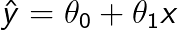Simple linear regression

Where x = feature value.

Ŷ = predicted value.

θ0 and θ1 collectively represent the parameters or coefficients in the model.

# Best fit Line

What does the best fit line mean? This means that the predicted value should be closer to the true value. It tends to minimize the error which is the difference between the true value and the predicted value.

# Multiple Linear Regression

Multiple linear regression tries to forecast the dependent variable using several independent variables.

Where θ is the vector of model parameters, contains bias term θ0 and model weights θ1 to θn.

# Cost Function

The cost function measures how well the model is performing on the training data set. Once the performance of the model is known, the model parameters can be adjusted to enhance the model’s functionality.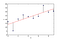Residuals

The residual or error is the distance between the true value and the predicted value. As the graph above shows the blue points are the true values, while the red line is the predicted values. And the distance between the red line and the blue points is the error or residuals.

## Mean squared error

The mean square error calculates the average of the squared distance between the true value and the predicted value. The lower the MSE value, the better the model performs.Mean squared error

Where N is the total number of observations.

## Root mean square error

The root-mean-square error is the square root of the mean squared error function. The RMSE function penalizes large outliers because it calculates the square root of the residuals before it averages them. Therefore, when there are large outliers in the data set, it is more useful to use the RMSE cost function.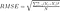Root mean square error

# Gradient Descent

Gradient descent is an optimization algorithm that updates the model parameters through an iterative process to minimize the cost function. You will better understand the gradient descent by a hypothetical scenario. Suppose on a foggy day you are standing on the mountain top and want to reach the bottom of the valley as soon as possible, what will you do in such conditions? You will feel the gradient of that place and head towards the negative gradient until you reach the valley. This is exactly what gradient descent does. It measures the local gradient of the cost function and moves towards the descending gradient until the gradient becomes zero.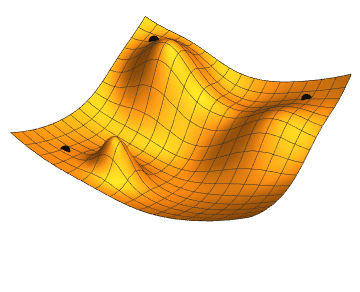Gradient descent

An important parameter of gradient descent is the learning rate, which determines the step size on each iteration while minimizing the loss function. Since you have to choose the learning rate manually, thus if you take a tiny value, it will take much time to converge at a global minimum or it may freeze in between and never converge at a global minimum.

On the other hand, if you choose a larger value, the algorithm may skip the global minimum and never converge.

# Batch Gradient Descent

Batch gradient descent uses the entire training dataset at every iterative step to calculate the gradient. It quantifies the average of the gradient of all training examples and then uses it to update the model parameters. The batch gradient descent process is slow and computationally expensive because it calculates the gradient of the entire dataset. If the cost function is smooth and convex in shape, then it is almost certain that the algorithm will converge at a global minimum. On the flip side, if the cost function is not convex, rather it has plateaus and deep valleys in between, then the algorithm may be stuck at a local minimum. Hence, you need to avoid using Batch gradient descent on larger data sets.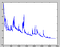Stochastic Gradient descent

# Stochastic Gradient Descent

Stochastic gradient descent selects a random instance in each step and then calculates the gradient. It reaches the global minimum faster than batch gradient descent, because there is very little data to be processed in each step. Owing to random nature, the cost function is very irregular, it never settles down instead of bounces up and down around the global minimum. Hence, the parameter values are good, but they are not optimal.

# Mini Batch Gradient Descent

Mini batch gradient descent finds a balance between Batch gradient descent and stochastic gradient. It splits the dataset into several small batches and then updates the model parameters by calculating the gradient of small batches. The advantage of using mini-batch gradient descent is that it uses matrix operations to speed up the calculation. Since we are using mini-batches in the training process, the fluctuation of the cost function is a bit like stochastic gradient descent. However, compared with the stochastic gradient, it reaches closer to the global minimum.

# Normal Equation

A normal equation is a method to reduce the cost function θ by calculating the coefficient of the multivariate regression. Contrary to Gradient Descent, the Normal equation is a one-step learning algorithm where you calculate θ directly.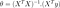Normal Equation

## Pros

1. There is no need to choose the learning rate in the normal equation.
2. The normal equation does not iterate through the data set to minimize theta.
3. This is a timesaving approach for small data sets.

## Cons

1. Slow if the number of features is very large in the dataset.

# Regularized Linear Models

Before we delve into regularization, you should understand what over-fitting is. When the model learns from the noise and incorrect data entries in the data set, the problem of over-fitting occurs. To overcome this problem, we use regularization in machine learning models.

## Ridge Regression

Ridge regression deals with the problems of multicollinearity and model complexity. It adds the L2 regularization to the cost function in order to minimize the model parameters’ weight.

α controls the regularization of the model. If the value of the hyper-parameter α is 0, the model is just linear regression. But as the value of α increases together, the weights of the model shrinks, and the model becomes much simpler. Before performing regularization, you must scale the data because Ridge regression is sensitive to the scale of the input features.

## Lasso Regression

Lasso regression is another form of linear regression, very similar to Ridge regression. It adds an L1 regularization to the cost function. The biggest difference between Ridge and Lasso regression is that in Ridge regression, the weights are close to zero, while in Lasso regression, some weights are reduced to absolute zero. Whenever the weight of a particular coefficient becomes zero Lasso regression obliterates that feature from the dataset. Therefore, feature selection can be done with the help of a Lasso regression.

## Elastic Net

The elastic net is the combination of both L1 and an L2 regularization term. You can think of it as a middle ground between the two regularization algorithms. If the value of the hyper-parameter α is 0, the behavior of the model is like that of the Ridge regression, but if α = 1, the behavior of the model is similar to that of the Lasso regression.

When the number of features in the data set exceeds the number of training instances, you should consider using elastic net over lasso regression.

## More from Shubham singh

Linkedin profile - https://www.linkedin.com/in/shubham-singh-112b161b9/